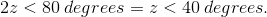# High School Math : Acute / Obtuse Isosceles Triangles

## Example Questions

← Previous 1

### Example Question #1 : How To Find The Height Of Of An Acute / Obtuse Isosceles Triangle

An isosceles triangle has a base of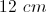and an area of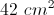. What must be the height of this triangle?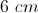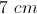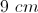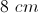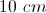Explanation: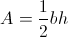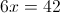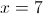### Example Question #1 : Isosceles Triangles

One side of an acute isosceles triangle is 15 feet.  Another side is 5 feet.  What is the perimeter of the triangle in feet?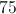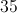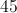Explanation:

Because this is an acute isosceles triangle, the third side must be the same as the longer of the sides that you were given.  To find the perimeter, multiply the longer side by 2 and add the shorter side.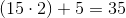### Example Question #2 : Isosceles Triangles

An isoceles triangle has a base angle five more than twice the vertex angle.  What is the difference between the base angle and the vertex angle?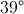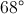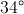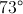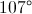Explanation:

A triangle has 180 degrees.  An isoceles triangle has one vertex angle and two congruent base angles.

Let= vertex angle and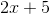=  base angle

So the equation to solve becomes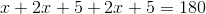or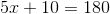So the vertex angle is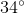and the base angle is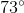so the difference is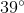### Example Question #2011 : Hspt Mathematics

Points A and B lie on a circle centered at Z, where central angle <AZB measures 140°. What is the measure of angle <ZAB?

Cannot be determined from the given information

20°

25°

15°

30°

20°

Explanation:

Because line segments ZA and ZB are radii of the circle, they must have the same length. That makes triangle ABZ an isosceles triangle, with <ZAB and <ZBA having the same measure. Because the three angles of a triangle must sum to 180°, you can express this in the equation:

140 + 2x = 180 --> 2x = 40 --> x = 20

### Example Question #2012 : Hspt Mathematics

Triangle FGH has equal lengths for FG and GH; what is the measure of F, if G measures 40 degrees?

100 degrees

70 degrees

140 degrees

40 degrees

70 degrees

Explanation:

It's good to draw a diagram for this; we know that it's an isosceles triangle; remember that the angles of a triangle total 180 degrees.

Angle G for this triangle is the one angle that doesn't correspond to an equal side of the isosceles triangle (opposite side to the angle), so that means F = H, and that F + H + 40 = 180,

By substitution we find that F * 2 = 140 and angle F = 70 degrees.

### Example Question #2013 : Hspt Mathematics

The vertex angle of an isosceles triangle is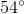.  What is the base angle?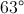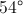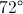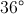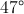Explanation:

An isosceles triangle has two congruent base angles and one vertex angle.  Each triangle contains.  Let= base angle, so the equation becomes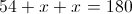.  Solving forgives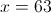### Example Question #2014 : Hspt Mathematics

In an isosceles triangle the base angle is five less than twice the vertex angle.  What is the sum of the vertex angle and the base angle?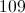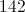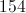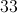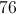Explanation:

Every triangle has 180 degrees.  An isosceles triangle has one vertex angle and two congruent base angles.

Let= the vertex angle

and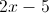= base angle

So the equation to solve becomes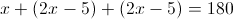or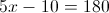Thus the vertex angle is 38 and the base angle is 71 and their sum is 109.

### Example Question #2015 : Hspt Mathematics

Sides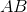and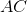in this triangle are equal. What is the measure of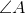?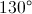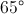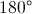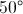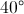Explanation:

This triangle has an angle of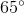. We also know it has another angle ofat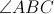because the two sides are equal. Adding those two angles together gives us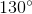total. Since a triangle hastotal, we subtract 130 from 180 and get 50.

### Example Question #3 : Isosceles Triangles

An isosceles triangle has a vertex angle that is twenty degrees more than twice the base angle.  What is the sum of the vertex and base angles?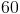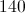40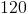Explanation:

All triangles containdegrees.  An isosceles triangle has one vertex angle and two congruent base angles.

Let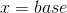and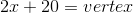.

So the equation to solve becomes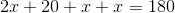.

We get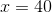and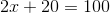, so the sum of the base and vertex angles is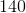.

### Example Question #4 : Isosceles Triangles

If an isosceles triangle has an angle measuring greater than 100 degrees, and another angle with a measuringdegrees, which of the following is true?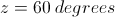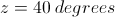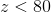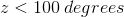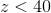In order for a triangle to be an isosceles triangle, it must contain two equivalent angles and one angle that is different. Given that one angle is greater than 100 degrees: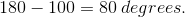Thus, the sum of the other two angles must be less than 80 degrees. If an angle is represented by: